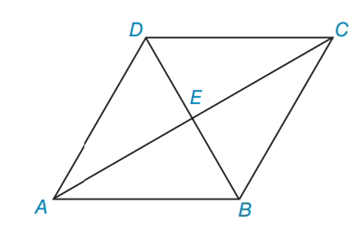Chapter 4.3, Problem 17EElementary Geometry For College St...

7th Edition
Alexander + 2 others
ISBN: 9781337614085

Solutions

Chapter
SectionElementary Geometry For College St...

7th Edition
Alexander + 2 others
ISBN: 9781337614085
Textbook Problem

In Exercises 15 to 18, consider rhombus ABCD with diagonals A C ¯  and  D B ¯ . When the answer is not a whole number, leave a square root answer.Exercises 15 – 18If AC = 10 and DB = 6, find AD.

To determine

To find:

AD of the given rhombus.

Explanation

The basic properties of rhombus:

1) All the properties of a parallelogram apply (the ones that matter here are parallel sides, opposite angles are congruent, and consecutive angles are supplementary).

2) All sides are congruent by definition.

3) The diagonals bisect the angles.

4) The diagonals are perpendicular bisectors of each other.

Calculation:

Given: AC = 10 and DB = 6

Consider the rhombus.

From the property, in rhombus, two diagonals are perpendicular bisectors of each other.

In rhombus ABCD

AE=EC=AC2=102=5

DE=EB=D

Still sussing out bartleby?

Check out a sample textbook solution.

See a sample solution

The Solution to Your Study Problems

Bartleby provides explanations to thousands of textbook problems written by our experts, many with advanced degrees!

Get Started

Fill in each blank: 4qt1pt=pt

Elementary Technical Mathematics

let f(x) = x 1, g(x) = x+1, and h(x) = 2x3 1. Find the rule for each function. 9. f + g

Applied Calculus for the Managerial, Life, and Social Sciences: A Brief Approach

True or False: 23xdx is an improper integral.

Study Guide for Stewart's Single Variable Calculus: Early Transcendentals, 8th

In Exercises 15-28, construct a truth table for the compound proposition. (qp)

Finite Mathematics for the Managerial, Life, and Social Sciences